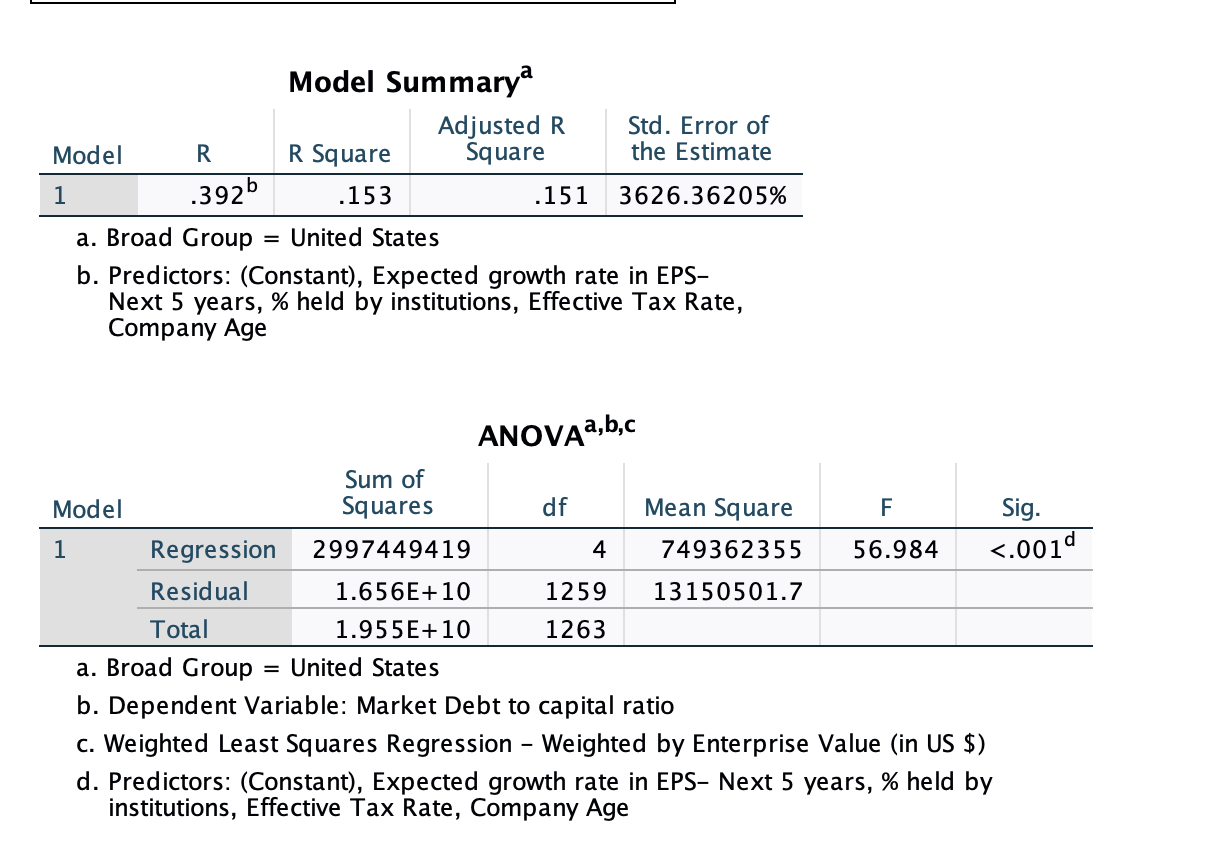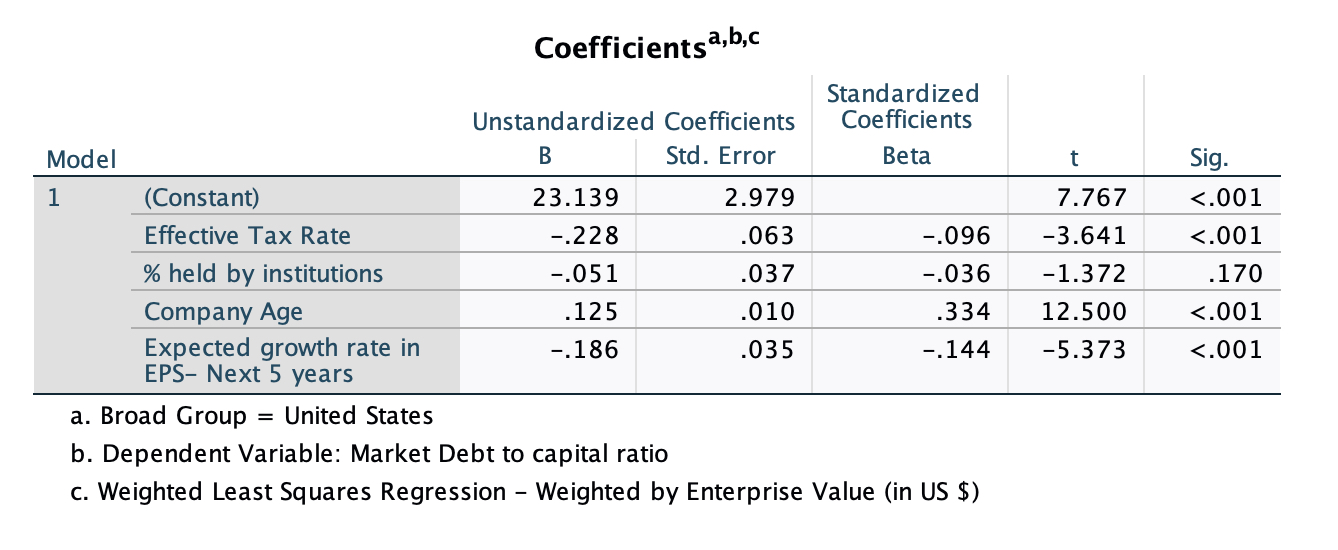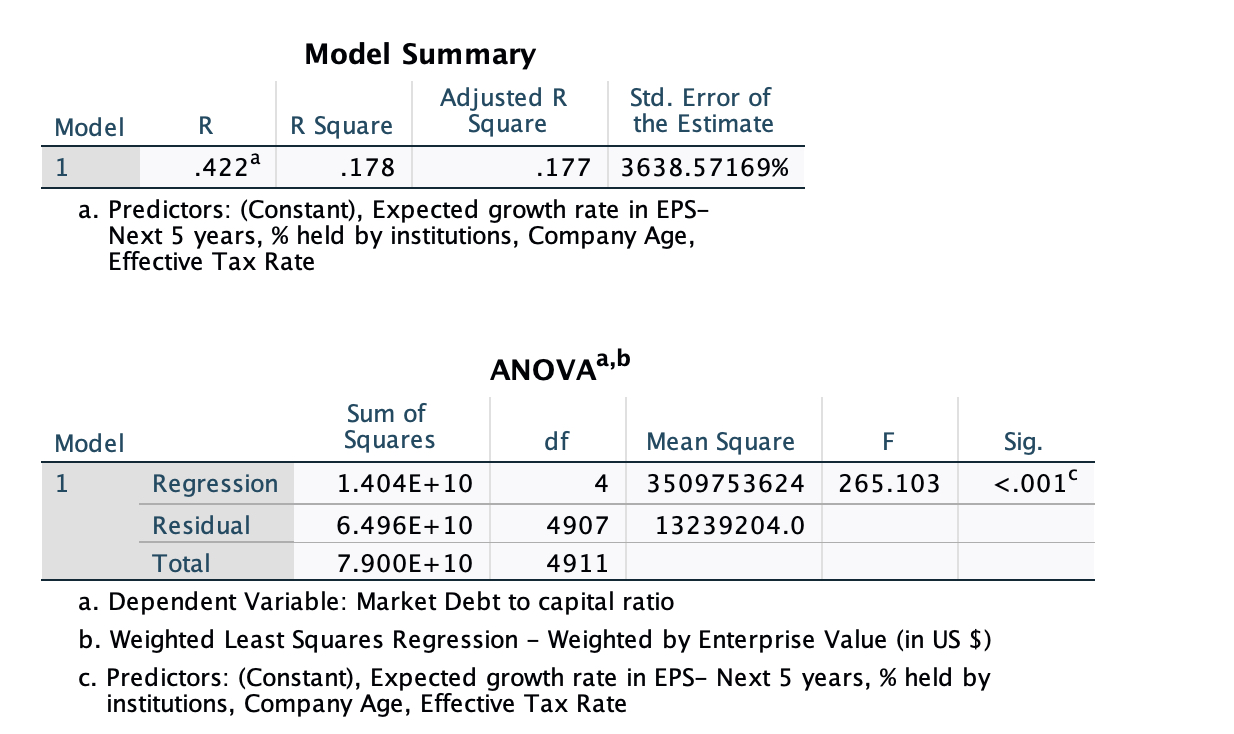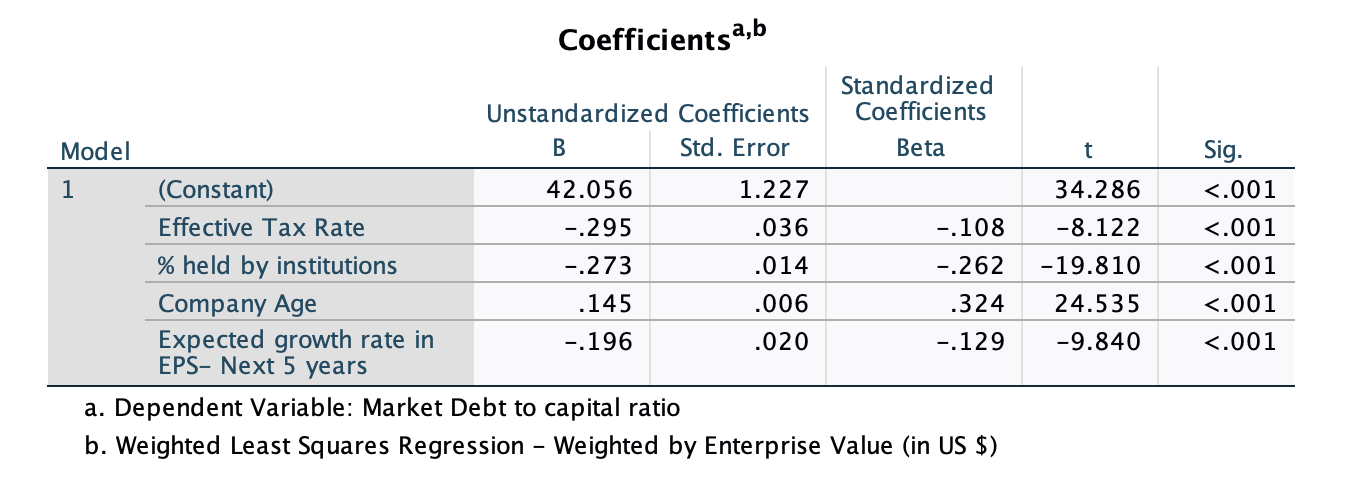##Debt Ratio Regression: January 2023

Variables used in the regression

1. Debt Ratio = Debt/ (Market Value of Equity + Debt): If you can get market value of debt, use it. Else, use book value of debt.
2. Payout Ratio= Dividends/ Net Income, if Net Income is positve, not available if net income is negative.
3. Expected growth rate in EPS- next 5 years= You can use expected or even historical earnings growth, if you don't have an EPS growth forecast
4. Effective Tax Rate = Effective tax rate in most recent year

US Regression####Global Regression####• How do I use this regression?

Assume that you want to estimate the market debt ratio for a firm with the following characteristics, using the Global regression

Effective tax rate = 25%

% held by institutions = 30%

Company age = 37

Expected growth rate in EPS = 12%

• Predicted Value

Expected Debt Ratio =  42.06 - 0.295 (25) - 0.273*(30)+ 0.145 (37) - 0.196 (12) = 29.51 or 29.51%

If your predicted value is less than zero, your predicted debt ratio is zero.